# Relation between magnetism and material creep

witu
TL;DR Summary
Presumption about relation between Bloch equation and solid/fluid volumetric strain rate equation.
Hello,

I am a civil engineer and have been working on concrete creep phenomenon for several years now. After reviewing a large amount of literature on related topics throughout this time, some interesting observations have emerged, and they have formed the idea that the material creep mechanism has some connection with NMR (Nuclear Magnetic Resonance). This is just my presumption and I do not have a hundred percent certainty on this, because I do not have enough competence in magnetism theories, but I still want to justify this my presumption somehow.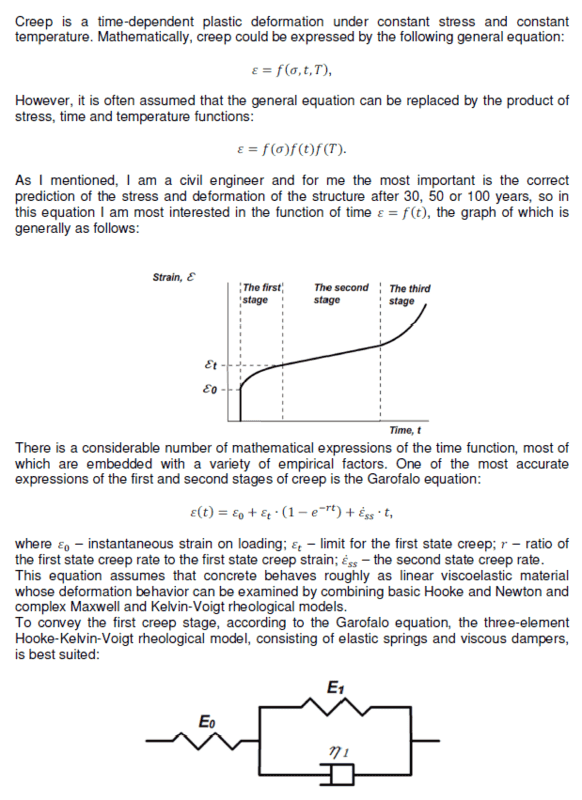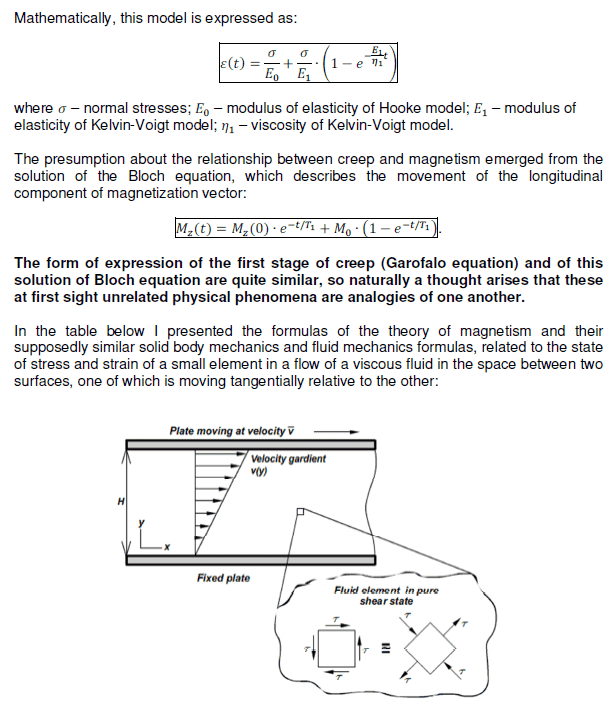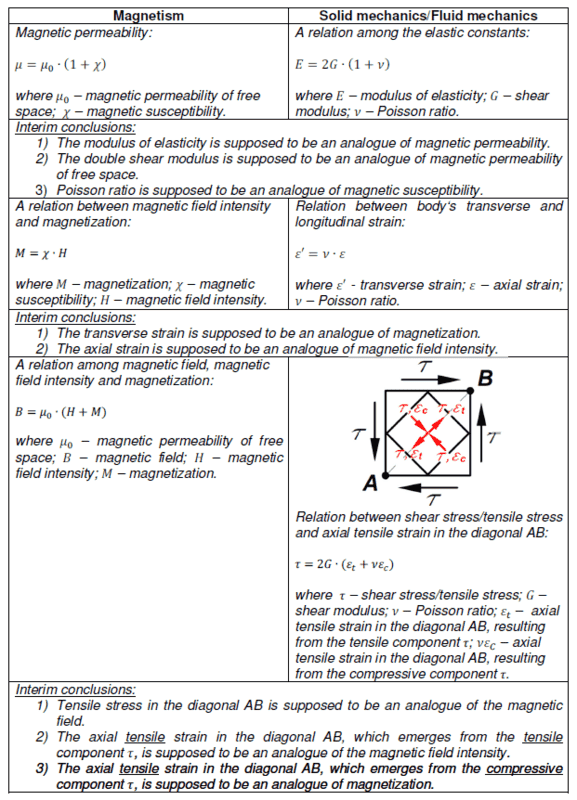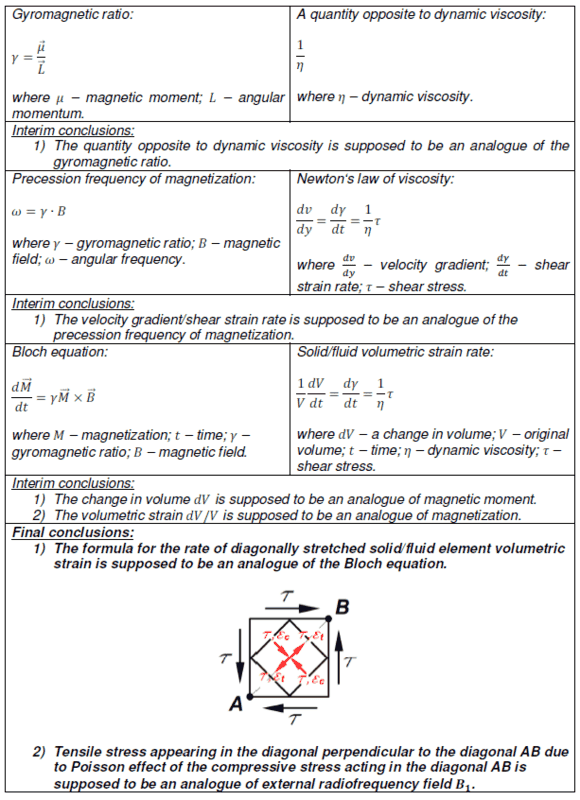•trurle

trurle
Bloch equation is empirical (no firm theoretical proof for it). Same applies to creep equation. Similarity of form may indicate in both cases maker of equation have a similar math education background, and do not guarantee more precise forms of equations will still have a matching form.

Welcome to PF.
There is absolutely no evidence that material creep and magnetism are related.

The similarity of a mathematical forms is called an “analog”. For example;
Kinetic energy = half * mass * velocity squared;
Capacitive energy = half * capacitance * voltage squared;
Inductive energy = half * inductance * current squared.
https://en.wikipedia.org/wiki/Structural_analog_(electronic)
Correlation does not imply causation.
https://en.wikipedia.org/wiki/Correlation_does_not_imply_causation

•anorlunda
witu
I agree, I also find it odd to associate these two different things, but let's say there is a phenomenon like the Villari effect which links stresses and magnetization. This effect prompted me to use the term "relation".
Okay, apparently I used the wrong term. The term "analog" is also suitable for me. Below I will describe why I do all this.
I am using DEM (Discrete Elements Method) to simulate the compaction of particles in a given volume. My desire is to characterize each particle somehow uniquely in this particle collection.
I did so: until the movement of the particles in the volume stopped, I represented the change of the speed vector position for each particle.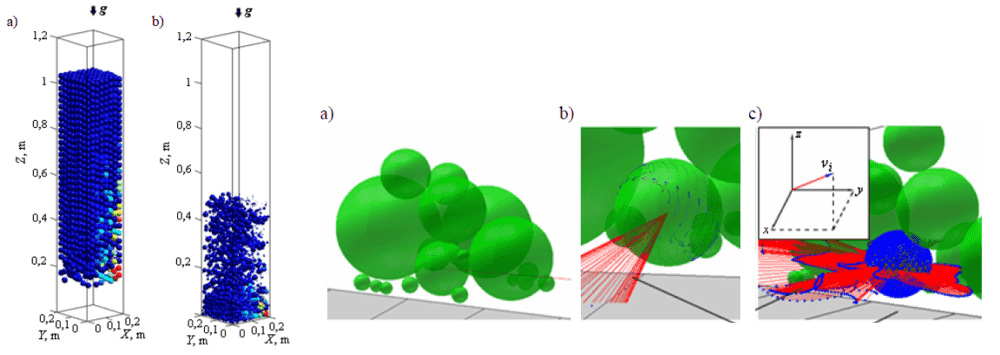As a result, certain characteristic textures were formed on the surface of the particles: the particles at the top of the volume in question were simply rotating and collinear circles formed on their surface, and the trajectory of the most compressed particles at the bottom of the volume in question received a set of "8" trajectories forming a diamond texture. The movement of these diamond-like particles resembled Rabi oscillations.After trying to graphically represent the change in the angle of the particle velocity vector, I received spiral graphs similar to free induction decay (FID) in Fourier transform nuclear magnetic resonance spectroscopy.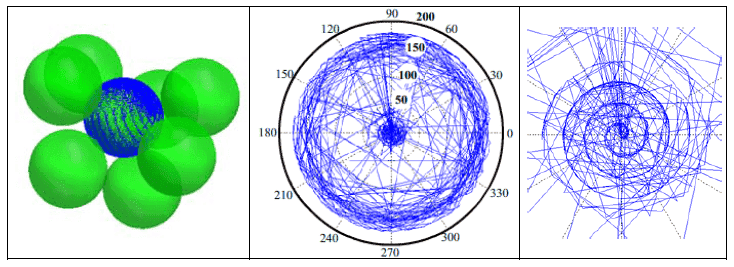After all, there is a big surprise when you perform a simple experiment of free fall of particles, but you see all the physics in it and after that, the strangest questions arise :)

•